# Fractions - math word problems

1. Water reservoirThe water reservoir is filled with one pump for 4 days by the second one for 9 days. Outlet can drain tank in 12 days. How long take fill the reservoir if both pumps are running and not closed outlet channel.
2. Two numbersThe sum of two numbers is 1. Identify this two numbers if you know that the half of first is equal to the third of second number.
3. PointsGryffindor won 437 points. How many points obtained by each of the faculties if they were split at a ratio of 5: 7: 3: 4?
4. Bridge piers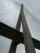One quarter of the bridge pier is sunk into the ground. Two thirds are in the water. Protruding above the water is 1.20 m long. Determine the height of bridge piers.
5. Roses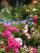On the large rosary was a third white, half red, yellow quarter and six pink. How many roses was in the rosary?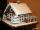Janka and Marienka calculated that there are 210 gingerbreads on the gingerbread house. Janko ate one-seventh of all gingerbreads, and Marienka ate a third less than Janko. How many gingerbreads remained in the gingerbread house?
7. Land areaA land area of Asia and Africa are in a 3: 2 ratio, the European and African are is 1:3. What are the proportions of Asia, Africa, and Europe?
8. Disinfecting solutionHow much distilled water is necessary to pour into 500 ml of 33% hydrogen peroxide solution to obtain 3% disinfecting solution?
9. Division of moneyCalculate how many euros have Matthew, Miriam, Lucy, Michael, Janka when together have 2,700 euros and the amounts are at a ratio of 1:5:6:7:8.
10. NumberI think the number. If I add to its third seven I get same as when to its quarter add 8. Which is the number?
11. Boots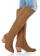Master shoemaker has three apprentices. First do one pair of boots for two days, second for 1 day, third on 1.5 day. If they worked together, for how long it would take made a couple of boots?
12. Cyclist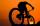Cyclist rode two-fifths of the way the first day. The second day drove 5 km less while traveled three-eighths of route. How many kilometers does he have to finish target?
13. Trees3/5 trees are apples, cherries are 1/3. 5 trees are pear. How many is the total number of trees?
14. Master and apprentice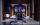Master painted the roof in 3 hours and apprentice for 4 hours. How many of roof they painted in hour and how many in three quarters of an hour?
15. Water in body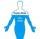Water in the human organism of all the compounds represented, constitutes about 3/5 of the total weight. How many kilograms of water is in your body when you weigh 67 kg?
16. Three monksThree medieval monks has task to copy 600 pages of the Bible. One rewrites in three days 1 page, second in 2 days 3 pages and a third in 4 days 2 sides. Calculate for how many days and what day the monks will have copied whole Bible when they begin Wednes
17. Divide moneyDivide 1200 USD at a ratio of 1:2:3:4:5:6:9:10
18. Mr. Happy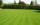Mr. Happy planted 36.6 meters square gardens grass; It's a third of the garden more than half of the garden. What is square area of this garden?
19. Container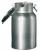How many three-quarters liters jar fill 30 liters container?
20. Pumps 5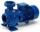5 pump pumped in 3 hours 1800 hl of water. How many hectoliters of water pumped 4 equally powerful pumps in 6 hours?

Do you have an interesting mathematical word problem that you can't solve it? Submit math problem, and we can try to solve it.

We will send a solution to your e-mail address. Solved examples are also published here. Please enter the e-mail correctly and check whether you don't have a full mailbox.

Please do not submit problems from current active competitions such as Mathematical Olympiad, correspondence seminars etc...

Need help calculate sum, simplify or multiply fractions? Try our fraction calculator.